# How to Remove Text in Excel Starting From a Position in Excel

In this article, we will learn how you can remove a number of characters from a string from a starting position.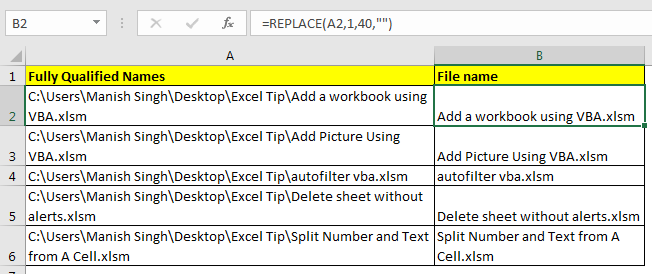The excel REPLACE function gives a us the functionality to replace number of characters from a string to with another string.

Generic Formula to Remove Text From a Position

=REPLACE(string, start_position, num_of_chars, “”)

String: this is the original string from which you want to remove characters.

Start_position: starting position in string.

Num_of_chars: number of characters you want to remove.

“” (blank): Replaces given characters from nothing. Ultimately removing the text.
Example 1: Remove Text From a String with Fixed Length.
Here I have list of fully qualified names of .xlsm files. I just want have only file’s name in list and remove every thing else.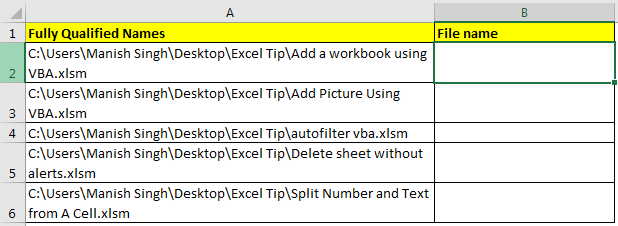Since “C:\Users\Manish Singh\Desktop\Excel Tip\” is the parent folder of each file and it is of 40 characters, we will write this formula to remove this common text from string.

=REPLACE(A2,1,40,"")

This excel formula removes 40 characters starting from first character.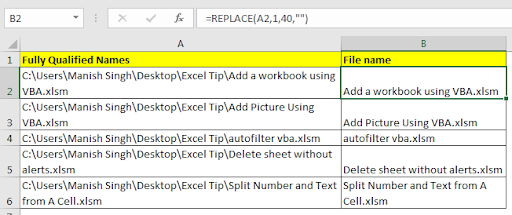Example 2: Remove Text From a String with Variable Length
In above example the length of text was fixed for removing. If the length of text which need to be removed is not fixed than take help of excel SEARCH function. This function returns the position of found text.

The above example was from one computer. If the data was compiled from different users then the structure of file path would be different. Only common thing will be the main directory, which is set to be name common. In my example it is Excel Tip.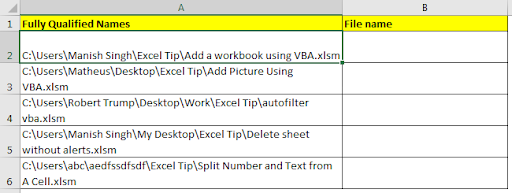So now to remove non required text from string write this formula.

=REPLACE(A2,1,SEARCH("Excel Tip\",A2)+9,"")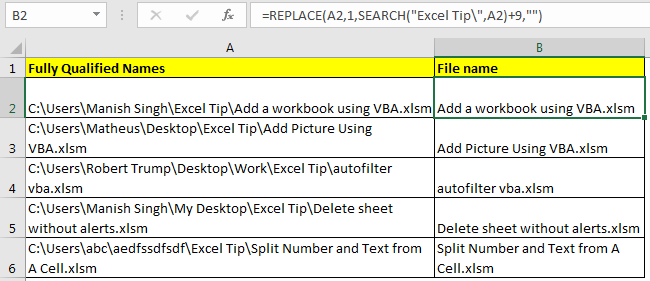How did it work?

Let’s start from inside.SEARCH("Excel Tip\",A2)+9: the search part will return the position of “Excel Tip\” in A2. which is 23. Now since it is the position of starting "Excel Tip\" in the string. We need the ending position of the text. Since “excel tip/” is a total of 10 characters we added 9 to get an accurate number. Hence we get 32.

Now the formula translates to REPLACE(A2,1,32,""). This formula removed non required text from string.

Same happens with all the strings.

So yeah guys, this is how you can remove text from the string in excel. Note that this tutorial was about removing text not extracting text in excel. However, both are very similar tasks in excel. You can find extraction tutorials below.

Popular Articles

50 Excel Shortcut to Increase Your Productivity: Get faster at your task. These 50 shortcuts will make you work even faster on Excel.

How to use the VLOOKUP Function in Excel: This is one of the most used and popular functions of excel that is used to lookup value from different ranges and sheets.

How to use the COUNTIF function in Excel: Count values with conditions using this amazing function. You don't need to filter your data to count specific values. Countif function is essential to prepare your dashboard.

How to use the SUMIF Function in Excel: This is another dashboard essential function. This helps you sum up values on specific conditions.

Terms and Conditions of use

The applications/code on this site are distributed as is and without warranties or liability. In no event shall the owner of the copyrights, or the authors of the applications/code be liable for any loss of profit, any problems or any damage resulting from the use or evaluation of the applications/code.